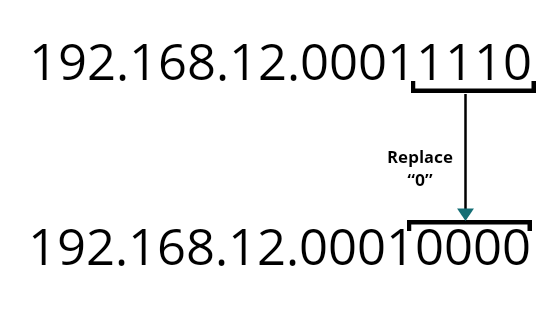# Classless Addressing in Computer Network

Computer Network | Classless Addressing: In this tutorial, we will learn about the classes addressing in computer network with their properties, representation, and examples. By Anshuman Das Last updated : May 04, 2023

To reduce the wastage of IP addresses in blocks we subnetting. But in Classless addressing wastage of IP addresses in a block is more reduced than Classful subnetting. In this variable length, blocks are used that belongs to no class.

1. Addresses in a block must be in contiguous form
2. The number of address in a block must be the power of 2 i.e. 2, 4, 8, 16,...
3. The first address must be evenly divisible by the number of addresses.

In Classless addressing a block, IP address is given like 192.168.10.1/28 (after "/" number of the mask bit is given).

We can find a mask for the whole block by putting the given after of bits out of 32 as 1 and rest of the bits as 0.

Here, we have 28 bits. So, we need to put 28 bits out of 32 bits as 1 and rest of bits as 0 will give us the mask for the Ip address block.

```    11111111.11111111.11111111.11100000
255.     255.     255.     240
```

Note: The given address is not necessarily the first IP address of the block.

Important points:

1. To get the first IP address of the block set the rightmost (32 - n) bits to 0s.
2. Last IP address of the block can be found by setting the rightmost bits to 1s.
3. Number of IP addresses of the given block can be found by 232 - n.

Example:

```    192.168.12.30/28
```

In the above example, if we want to find the first address of the given block then have to put 0 to set a rightmost bit of the given IP.

To make is easy to convert only the last octet into binary and then set 1 or 0 accordingly and rest will remain the same.

Binary of 30 = 11110Hence, the first IP address of the block is 192.168.12.16 (Satisfying Property no. 3).

Again, to get the last IP address of the block we have to replace all the rightmost bit to 1, 192.168.12.00011110

After replacing all the rightmost bits to 1 we obtain 192.168.12.00011111 i.e. 192.168.12.31

Suggestion: To understand this topic please go through the previous topic i.e. FLSM and VLSM in Computer Networks